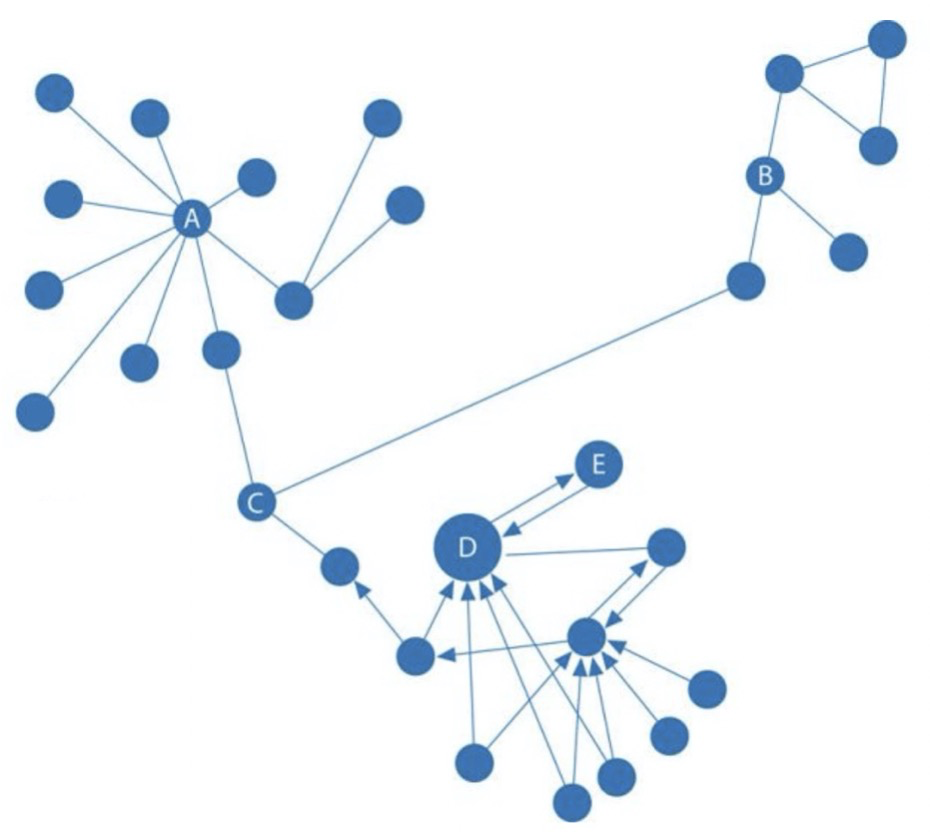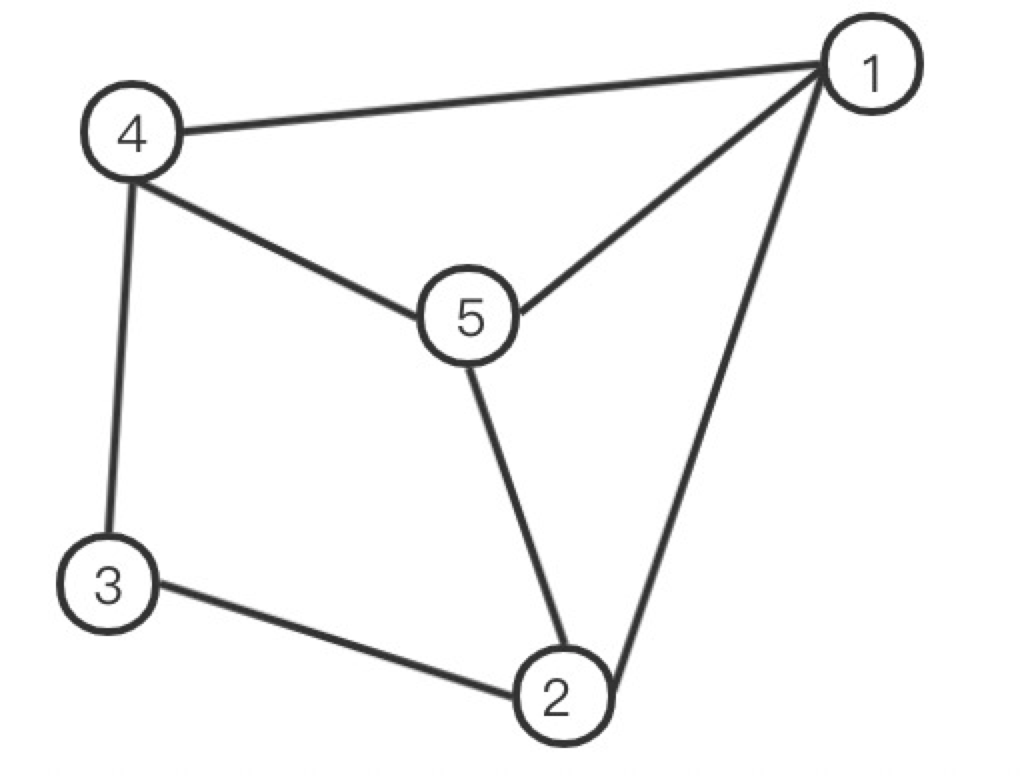# 基于 NebulaGraph 的 Betweenness Centrality 算法​在图论中，介数（Betweenness）反应节点在整个网络中的作用和影响力。而本文主要介绍如何基于 NebulaGraph 图数据库实现 Betweenness Centrality 介数中心性的计算。

## 2. 应用场景## 3. 介数中心性公式

$$C_B = \sum_{s{\not=} v {\not=} t \in V} \frac{\sigma_{st}(v)}{\sigma_{st}}$$

（公式 1）

$$\sigma_{st}(v)$$：经过节点 v 的 s 到 t 的最短路径条数； $$\sigma_{st}$$：节点s到节点t的所有最短路径条数；

s 和 t 是属于节点集合的任意一个节点对。

$$\delta_{st}(v) = \frac{\sigma_{st}(v)}{\sigma_{st}}$$

（公式 2）

$$C_B(v) = \sum_{s{\not=} v {\not=} t \in V} \delta_{st}(v)$$

（公式 3）

## 4. 求解思路

（1）求节点 v 是否在 s 到 t 的最短路径上，采用下面公式判断($$d_G$$ 表示两点之间的最短路径长度)：

（公式 4）

$$\sigma_{st}(v) = \begin{cases} \sigma_{sv} \times \sigma_{vt} &\text{if } d(s,v) + d(v,t) = d(s,t) \ 0 &\text{if } other \end{cases}$$

（公式 5）

（2）根据上面公式可得：

$$\sigma_{st}(v,{v,w}) = \sigma_{sv} \times \sigma_{wt}$$

（公式 6）

（一）$$t = w$$ 时，不存在$$\sigma_{wt}$$

$$\delta(v,{v,w}) = \frac{\sigma_{sv}}{\sigma_{sw}}$$

（公式 7）

（二）$$t \not= w$$ 时

$$\delta(v,{v,w}) = \frac{\sigma_{sw}(v)}{\sigma_{sw}} \times \frac{\sigma_{st}(w)}{\sigma_{st}}$$

（公式 8）

（3）所以将上面两种情况加起来，得到经过 v 的 s 到所有顶点的最短路径数占 s 到所有顶点的最短路径数的比值。

$$\delta_s(v) = \sum_{w:v \in P_s(w)}(\frac{\sigma_{sw}(v)}{\sigma_{sw}} + \sum_{t \not= w \in V}\frac{\sigma_{sw}(v)}{\sigma_{sw}} \times \frac{\sigma_{st}(w)}{\sigma_{st}}) \ = \sum_{w:v \in P_s(w)}\frac{\sigma_{sw}(v)}{\sigma_{sw}}(1 + \sum_{t \not= w \in V} \frac{\sigma_{st}(w)}{\sigma_{st}}) \ = \sum_{w:v \in P_s(w)}\frac{\sigma_{sw}(v)}{\sigma_{sw}} (1 + \delta_s(w))$$

（公式 9）

（4）根据上面的求 $$\delta_{sv}$$ 的公式，下面给出论文中求解无权图时的算法流程，如下所示。## 5. 计算示例2-4 3 （2-3-4，2-5-4，2-1-4） 1

1-3 2 （1-2-3，1-4-3） 1
3-5 2（3-2-5，3-4-5） 1

2-4 3 （2-3-4，2-5-4，2-1-4） 1

1-3 2 （1-4-3，1-2-3） 1
3-5 2（3-4-5.3-2-5） 1

2-4 3 （2-3-4，2-5-4，2-1-4） 1

## 6. 算法结果示例

(root@nebula) [test]> match (v:node) -[e:relation] -> ()  return e
+------------------------------------+
| e                                  |
+------------------------------------+
| [:relation "3"->"4" @1 {col: "f"}] |
+------------------------------------+
| [:relation "2"->"3" @2 {col: "d"}] |
+------------------------------------+
| [:relation "2"->"5" @4 {col: "e"}] |
+------------------------------------+
| [:relation "4"->"5" @2 {col: "g"}] |
+------------------------------------+
| [:relation "1"->"5" @1 {col: "a"}] |
+------------------------------------+
| [:relation "1"->"2" @3 {col: "b"}] |
+------------------------------------+
| [:relation "1"->"4" @5 {col: "c"}] |
+------------------------------------+


vid: 4 BC: 1.0
vid: 1 BC: 0.3333333333333333
vid: 3 BC: 0.3333333333333333
vid: 5 BC: 0.3333333333333333
vid: 2 BC: 1.0


vid: 4 BC: 2.0
vid: 1 BC: 0.5
vid: 3 BC: 1.0
vid: 5 BC: 2.0
vid: 2 BC: 0.0